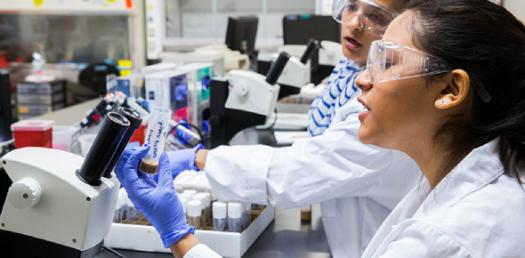# A Practice Test On Chemistry! Trivia Questions Quiz

25 Questions | Attempts: 149Settings.

• 1.
Particles of matter that make up the nucleus of the atom are ___.
• A.

Protons and neutrons

• B.

Protons and electrons

• C.

Electrons and neutrons

• D.

Ions and isotopes

• 2.
All of the mass of the atom is in the ___.
• A.

Nucleus

• B.

Electrons

• C.

Protons

• D.

Electron cloud

• 3.
Horizontal rows of the periodic table are called ___.
• A.

Periods

• B.

Clusters

• C.

Groups

• D.

Families

• 4.
Atoms of the same element with different numbers of neutrons are called ___.
• A.

Isotopes

• B.

Ions

• C.

Nucleates

• D.

Metalloids

• 5.
Elements that are gases, are brittle, and are poor conductors at room temperature are ___.
• A.

Non-metals

• B.

Metals

• C.

Metalloids

• D.

Actinides

• 6.
A certain atom has 38 protons, 38 electrons, and 50 neutrons. Its mass number is ___.
• A.

88

• B.

38

• C.

50

• D.

76

• 7.
Why are the noble gases stable?
• A.

They have a full outer shell of electrons

• B.

They are gases

• C.

They do not have any electrons

• D.

They were used by monarchies throughout history

• 8.
An atom that has gained 2 electrons and is now positively charged is specifically called a(n) ___.
• A.

Cation

• B.

Anion

• C.

Antion

• D.

Dogion

• 9.
Elements that fall along the "staircase" on the periodic table and have properties like metals and non-metals are called___.
• A.

Metalloids

• B.

Non-metals

• C.

Isotopes

• D.

Metals

• 10.
What period and group (in that order) is barium found in?
• A.

6, 1

• B.

5, 2

• C.

2, 6

• D.

6, 2

• 11.
Given the following information, Iodine - 128, how many electrons are present?
• A.

53

• B.

75

• C.

128

• D.

127

• 12.
An atom that has gained two additional electrons would have a charge of ___.
• A.

+2

• B.

-2

• C.

-6

• D.

None

• 13.
Argon is considered a noble gas and non-reactive. What makes argon such a stable atom?
• A.

8 electrons in outer shell

• B.

More positive thatn negative charge

• C.

More negative than positive charge

• D.

None of these

• 14.
An atom found in period 4, group 7 would be considered a non-metal.
• A.

True

• B.

False

• 15.
In an atom, the atomic number is useful because a physical science student can determine the number of protons and electrons.
• A.

True

• B.

False

• 16.
If Tellurium - 127 were to lose 4 neutrons, the new mass would be 122.
• A.

True

• B.

False

• 17.
Oxygen has 5 valence electrons.
• A.

True

• B.

False

• 18.
Sodium has 11 electrons total, but only 2 electrons in its first energy level.
• A.

True

• B.

False

• 19.
The elements on the modern Periodic Table are arranged by decreasing atomic number.
• A.

True

• B.

False

• 20.
Electrons farthest from the nucleus have a higher level of energy as compared to other electrons in the atom.
• A.

True

• B.

False

• 21.
Nuclear fusion occurs naturally in the sun.
• A.

True

• B.

False

• 22.
The three subatomic particles are ___.
• A.

Protons, neutrons, and electrons

• B.

Photons, ions, and isotopes

• C.

Nucleus, electrons, and photons

• D.

Protons, nucleons, and quarks

• 23.
The mass of an electron is so small, we sometimes say it's mass is negligible.
• A.

True

• B.

False

• 24.
Two isotopes of hydrogen are hydrogen-2 and hydrogen-3. These isotopes differ from one another by one proton.
• A.

True

• B.

False

• 25.
The region around the nucleus occupied by the electrons is called the negative zone.
• A.

True

• B.

False

## Related TopicsBack to top
×

Wait!
Here's an interesting quiz for you.